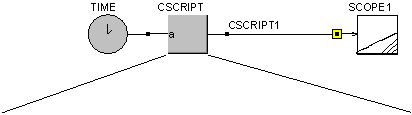• Introduction
• What is in this manual
• What is Caspoc
• User interface
• Introduction
• Starting
• Simulation
• Editing
• Viewing and printing
• Getting Started
• Basic editing
• Simulation in the time domain
• Basic User Interface Topics
• Editing
• Simulation
• Viewing
• Library
• Reports
• Project management
• Circuit and Block Diagram Components
• Introduction
• Cscript and user defined functions
• Component parameters
• Modeling Topics
• Introduction
• Power Electronics
• Semiconductors
• Electrical Machines
• Electrical drives
• Power Systems
• Mechanical Systems
• Thermal Systems
• Magnetic Circuits
• Green Energy
• Coupling to FEM
• Experimenter
• Analog hardware description language
• Embedded C code Export
• Coupling to Spice
• Small Signal Analysis
• Matlab coupling
• Tips and tricks
• Appendices

## Create a Cscript in the block diagram

For complex models or modeling a control the Cscript block allows you to create your own blocks. The function of the block is defined by ANSI-C code, see the appendix for the details on the syntax. The input for the block Cscript is "a" and is defined as a local "int" variable inside the scripts Main function.1 2 3 4 5 6 7 8   int iCount; int y; main()    {    iCount=10;    y=a+iCount;    return(y);    }   // Local declaration of iCount // Local declaration of y // Main function // Opening brace // Set iCount to 1 // Add iCount to the input a // Set the output of the block to y // Closing brace 

Local variable can be defied outside the main function as is done in lines 1 and 2. There has to be one main function with opening and closing braces, see lines 3,4 and 8. The variable a is defined as being the input of the block. It doesn�t have to be declared, as it is done automatically by Caspoc as a local variable that is defined inside the scope of the main function. The argument of the return function, in this case y in line 7, is the output of the block.

The blocks Cscript2, Cscript3, ... Cscript20 have more inputs labeled from "a" until "t"

Inside the Cscript block you can use loops like for, while do and if-then-else structures. Functions can be called recursively and you can pass local variables as function arguments. Variables are declared from the type "int".

Features of Cscript include:

 Parameterized functions with local variables Recursion The if statement The do-while, while, and for loops Integer and character variables Global variables Integer and character constants String constants (limited implementation) The return statement, both with and without a value A limited number of standard library functions Operators: +, -, *, / , %, <, >, <=, >=, ==, !=, unary -, unary +, *=, +=, -=, /=, &=, |= and ^= Support for && and || in comparisons Functions returning integers Comments // and /*          */